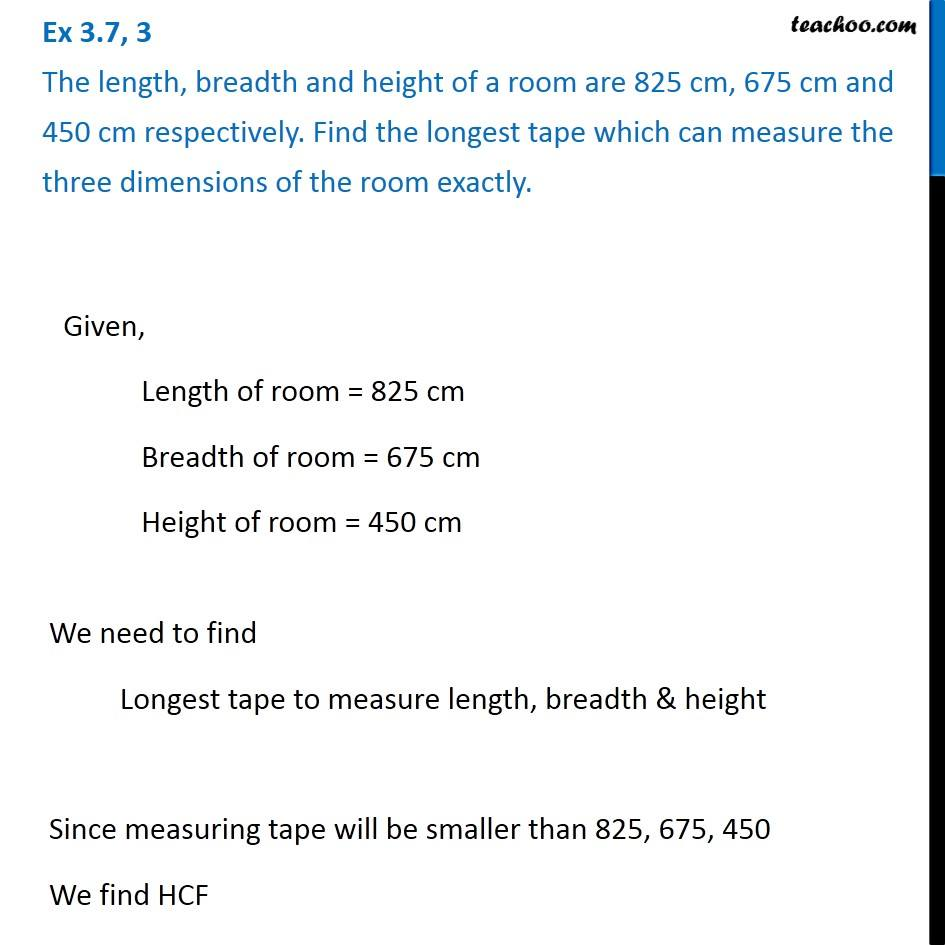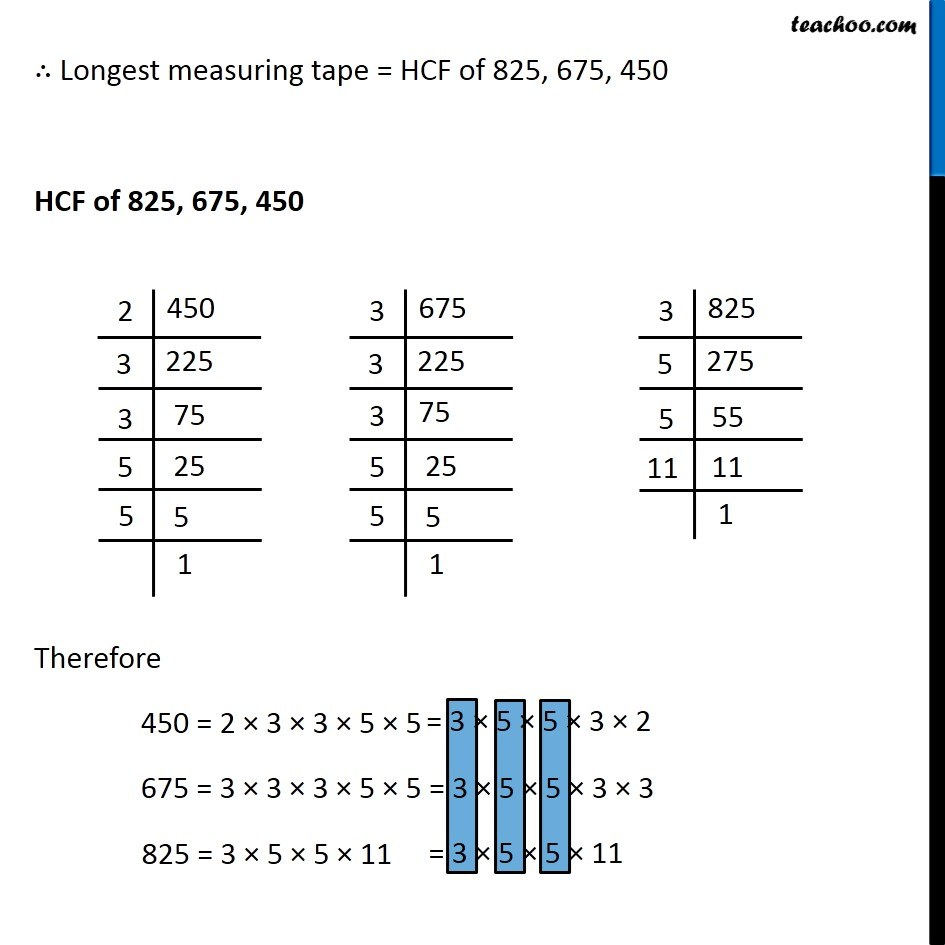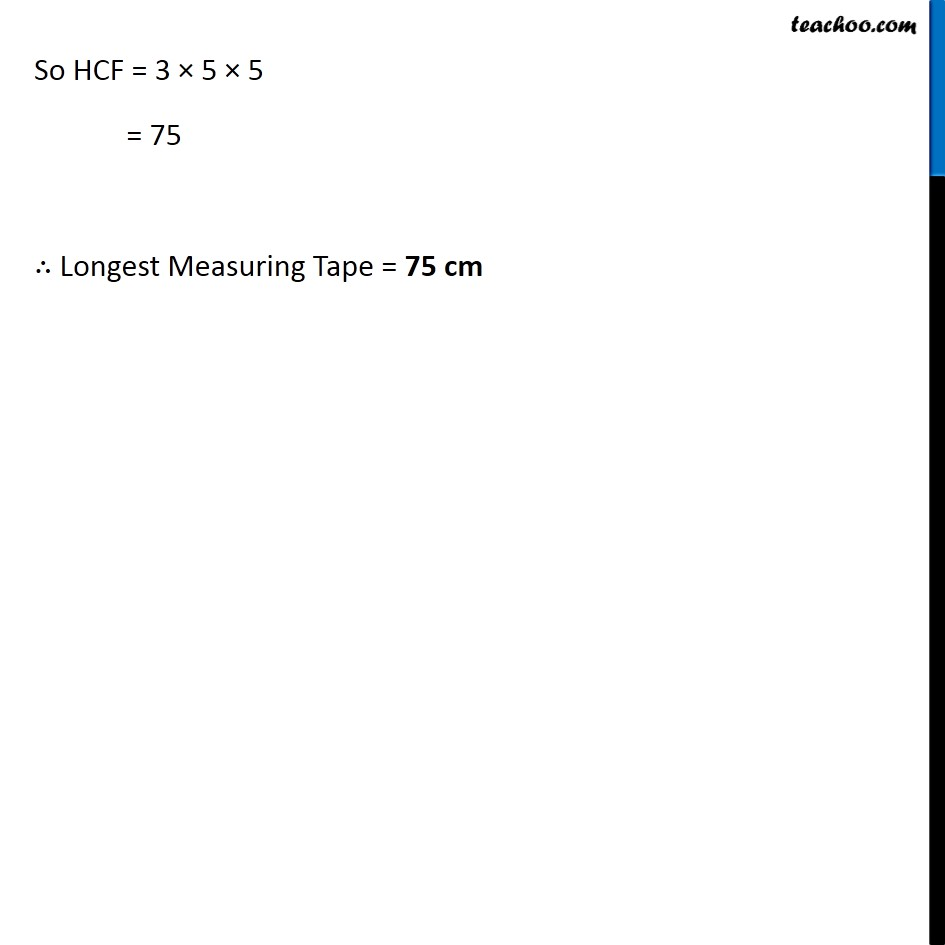Ex 3.7

Chapter 3 Class 6 Playing with Numbers
Serial order wiseLearn in your speed, with individual attention - Teachoo Maths 1-on-1 Class

### Transcript

Ex 3.7, 3 The length, breadth and height of a room are 825 cm, 675 cm and 450 cm respectively. Find the longest tape which can measure the three dimensions of the room exactly. Given, Length of room = 825 cm Breadth of room = 675 cm Height of room = 450 cm We need to find Longest tape to measure length, breadth & height Since measuring tape will be smaller than 825, 675, 450 We find HCF ∴ Longest measuring tape = HCF of 825, 675, 450 HCF of 825, 675, 450 Therefore 450 = 2 × 3 × 3 × 5 × 5 675 = 3 × 3 × 3 × 5 × 5 825 = 3 × 5 × 5 × 11 So HCF = 3 × 5 × 5 = 75 ∴ Longest Measuring Tape = 75 cm